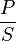# Power factor facts for kids

Kids Encyclopedia Facts

In electrical engineering, the power factor (PF or cosφ) is the ratio between the power than can be used in electric circuit (real power, P) and the power from the result of multiplication between the current and voltage circuit (apparent power, S). The power factor is defined as:$\frac{P}{S}$

PF ranges from zero to one. The higher the PF, the more efficient the circuit is because more power can be used, and vice versa. Factors that can cause PF to be less than one is non-linear load, which are capacitive load and inductive load.

## Images for kidsPower factor Facts for Kids. Kiddle Encyclopedia.